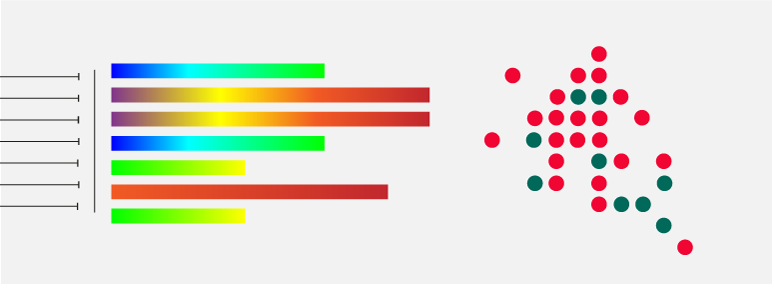After the pre-processing steps and optional quality check, it is possible to construct either supervised or unsupervised machine learning models. To avoid overfitting the models, dimensionality reduction is performed using principal component analysis (PCA) or a convolutional neural network (CNN). Depending on whether an identification or concentration determination is to be carried out, a classification or regression model is required. The following models are available in the software for this purpose:

Linear Discriminant Analysis (LDA);
Random Forest (RF);
k-nearest neighbours (kNN);
support vector machine (SVM);
partial least squares discriminant analysis (PLS-DA);
unsupervised hierarchical Ward cluster analysis (HCA);
linear regression;
partial least squares regression (PLSR).